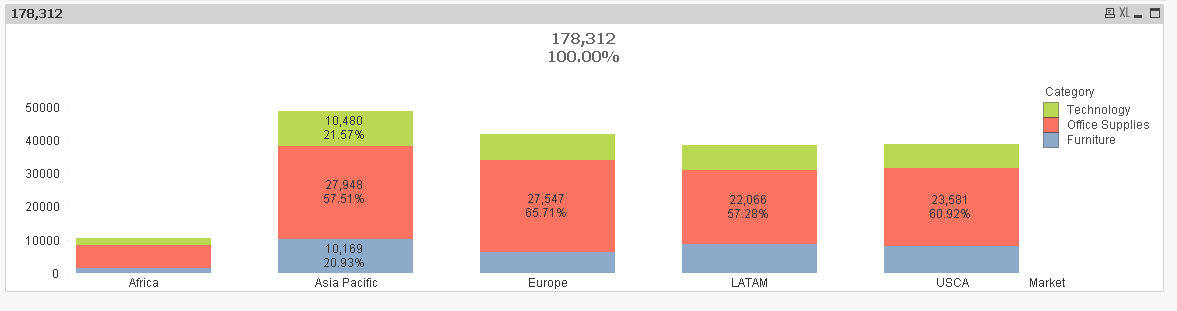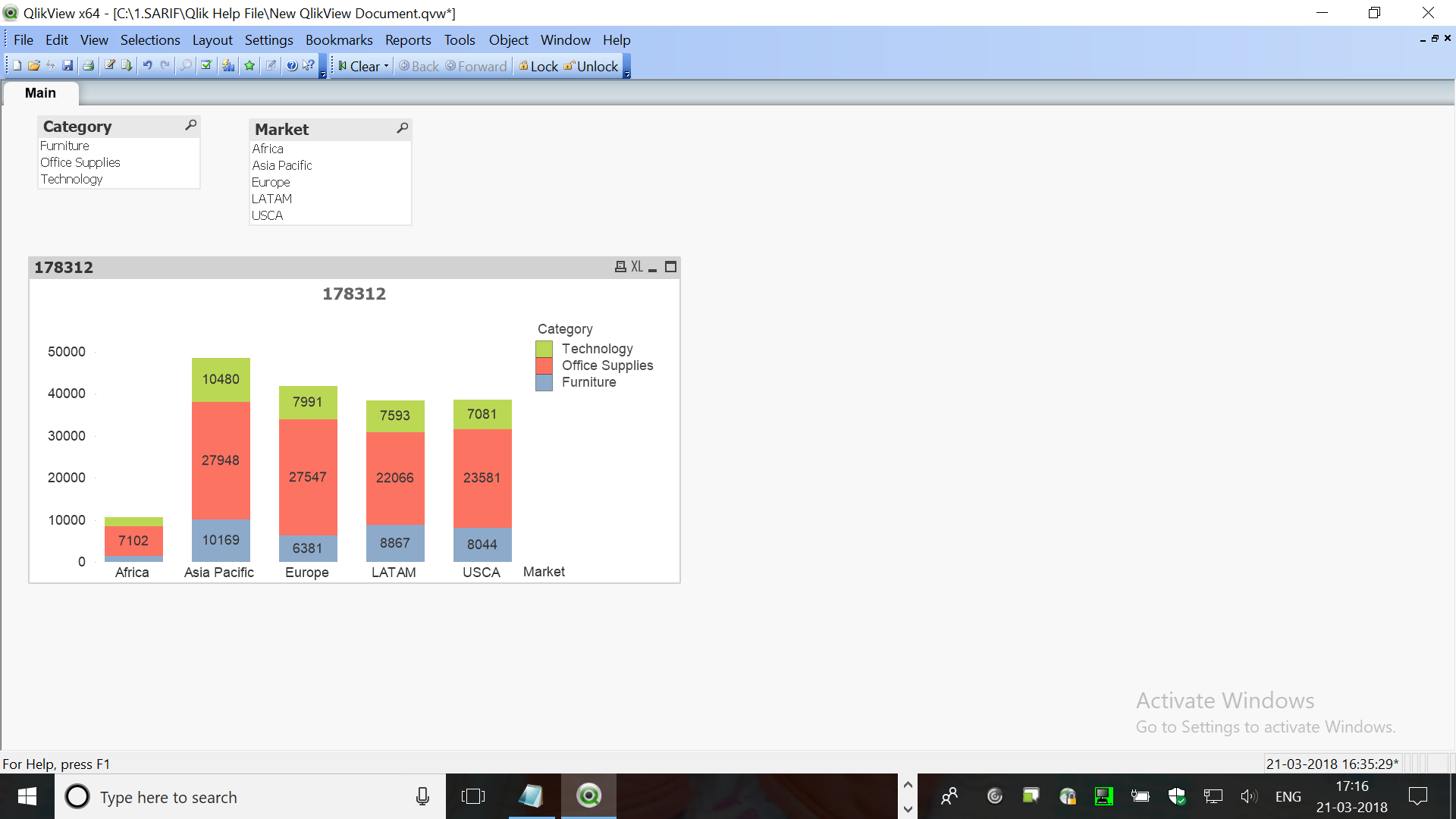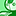# QlikView App Dev

Discussion Board for collaboration related to QlikView App Development.

Announcements
Welcome to Qlik Community! Check out our new navigation! FIND OUT MORE
cancel
Showing results for
Did you mean:Creator II

## Need Values and Percentage Share in Stacked bar Chart

Hi All,

I have a stacked bar chart on Category and Market.

I need Values as well as Percentage share on the bar chart.

Thanks,

Sarif

1 Solution

Accepted SolutionsMVP

Something like this?The only issue is that smaller bars won't really show any value because of the space constraints. Expression used

=Dual(Num(Sum(Quantity), '#,###.') & Chr(10) & Num(Sum(Quantity)/Sum(TOTAL <Market> Quantity), '##.##%'), Sum(Quantity))

14 RepliesMVP

One of these two?For one, use this expression

=Sum(Quantity)/Sum(TOTAL <Market> Quantity)

For two, check relative on the expression tabCreator II
Author

I need combination of Base and 1.

Chart should show you the vale and Percentage.Specialist II

PFA,

%age:

sum(Quantity)/SUm(TOTAL Quantity)

for 100% Staked bar:

sum(Quantity)/SUm(TOTAL <Market>  Quantity)MVP

Can you elaborate? What is Base?Partner - Creator III

than you will have to append both i supposeCreator II
Author

For below example:

Lets say for Asia Pacific.

I need to show values on data point Technology = 10480 along with this I want to show 21.56 % i.e. 10480/(10480 + 27948 + 10169)

So Sum and Percent both I need to show in Values on Data Point.MVP

Something like this?The only issue is that smaller bars won't really show any value because of the space constraints. Expression used

=Dual(Num(Sum(Quantity), '#,###.') & Chr(10) & Num(Sum(Quantity)/Sum(TOTAL <Market> Quantity), '##.##%'), Sum(Quantity))Creator II
Author

Thanks Sunny... this is I want.Creator

Hi Sunny,

will it work in Qliksense? I want to apply the same logic in Qliksense. I have created one cyclic group and I applied same logic which I have applied in Qlikview but not working in Qliksense. Is there any other way to achieve this in Qliksens?

Thanks & Regards,

Pandiarajan.R